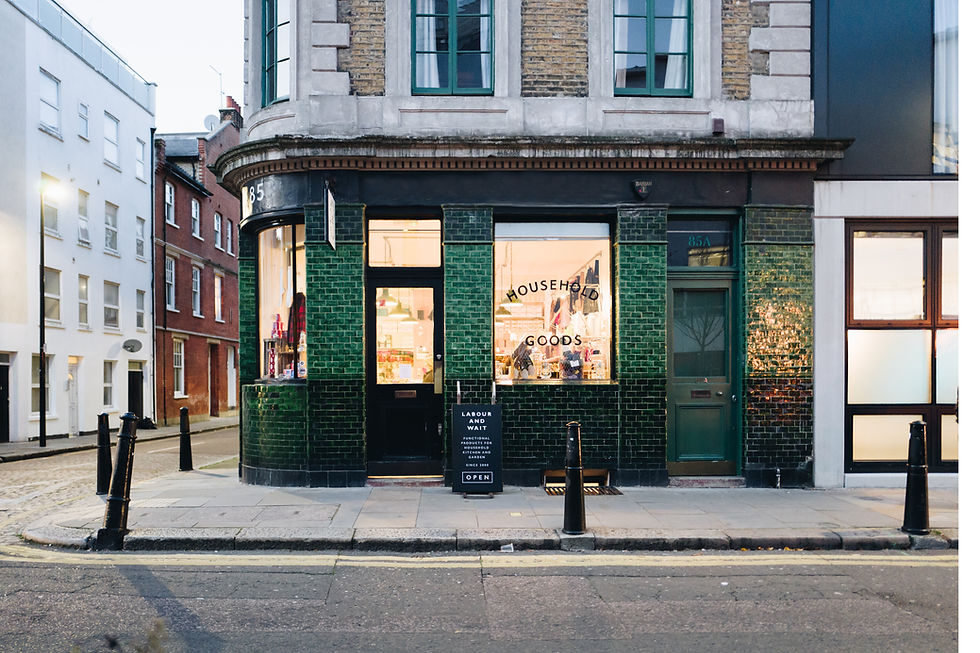top of page

# Final Project

Hooman is very rich. He has a plane, a ship, a scooter, a train, a bike, a car, a huaban, and a HSR.I use the acceleration sensor function to get the value, by determine the size of x and y to decide the direction of up , down , left and right, and then according to different directions to decide what kinds of transportation. In addition, I also make a design,  determine the size of x again to switch the pictures.## CODE

global u n k           % set the variables
n=3;
while(n==3)             % third while
n=1;
while(n==1)             % first while
clear all;
connector on
m= mobiledev;
pause(1);
imshow('hooman.jpg');
m.Logging= 1;               % start getting signal from cellphone
pause(2);
m.Logging=0;                 % stop getting signal from cellphone
[ac,tac] = accellog(m);      % accellog
xa = ac(:,1);                         % take value from x
ya = ac(:,2);                           % take value from y
x=xa(end);
y=ya(end);
assignin('base','x',x);
assignin('base','y',y);
sim('finalproject');
u=max(p);
if(u==1)
imshow('bike.jpg');
elseif(u==2)
imshow('scooter.jpg');
elseif(u==3)
imshow('huaban.jpg');
elseif(u==4)
imshow('car.jpg');
end
pause(2);
n=2;
end
while(n==2)                     % second while
clear all;
connector on
m= mobiledev;
pause(1);
imshow('ship.jpg');
m.Logging= 1;
pause(2);
m.Logging=0;
[ac,tac] = accellog(m);
xa = ac(:,1);
x=max(xa);
sim('speed');
k=max(s);
if(k==1)
imshow('train.jpg');
elseif(k==2)
imshow('hsr.jpg');
elseif(k==3)
imshow('air.jpg');
end
pause(2);
n=3;
end
endFinal Project: Image## Final Project Stateflow

Final Project: Image